##### The Japanese Bonsai specialist
Direct order Contact Help / Services Newsletter# Bonsai focus n° 53

Bonsai focus magazine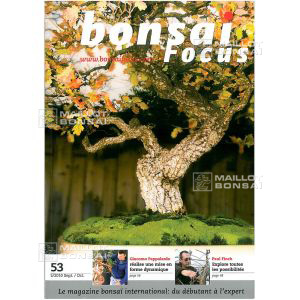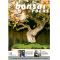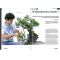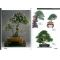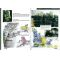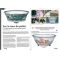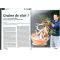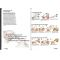ref. : 6651

9,95

Available quantity : 4Order

###### Description

This number will present you the history of Kariyushi, followed of a tree on several decades and put in shape by Masahiko Kimura.

You will be able to observe an astonishing landscape, in Germany, of spectacular rocky formations, by Wolfgang Kohlhepp, then.

Shinji Suzuki will help you in the choice and the preparation of the shelf.

Antonio Gesualdi will explain you a difficult case of which he should have coped, a stump without nebari.

Finally, Paul Finch will make you part of a formatting that takes an unexpected turn.

To finish, Giacomo Pappalardo will explain you a work achieves especially for Bonsaï Focus. "Ultimo" is a powerful Juniperus Sabina promised to a very brilliant future.

#will 6.4 #bonsai 3.6 #focus 3.5 #magazine 2.8 #explain 2.7 #spectacular 2.1 #preparation 2.1 #astonishing 2.1 #unexpected 2 #formatting 2

Formule
(( ROUND((CHAR_LENGTH(b.article_nom)-CHAR_LENGTH(REPLACE(b.article_nom, 'will', '')))/LENGTH('will')) + ROUND((CHAR_LENGTH(b.article_description)-CHAR_LENGTH(REPLACE(b.article_description, 'will', '')))/LENGTH('will')) ) * 6.4) + (( ROUND((CHAR_LENGTH(b.article_nom)-CHAR_LENGTH(REPLACE(b.article_nom, 'bonsai', '')))/LENGTH('bonsai')) + ROUND((CHAR_LENGTH(b.article_description)-CHAR_LENGTH(REPLACE(b.article_description, 'bonsai', '')))/LENGTH('bonsai')) ) * 3.6) + (( ROUND((CHAR_LENGTH(b.article_nom)-CHAR_LENGTH(REPLACE(b.article_nom, 'focus', '')))/LENGTH('focus')) + ROUND((CHAR_LENGTH(b.article_description)-CHAR_LENGTH(REPLACE(b.article_description, 'focus', '')))/LENGTH('focus')) ) * 3.5) + (( ROUND((CHAR_LENGTH(b.article_nom)-CHAR_LENGTH(REPLACE(b.article_nom, 'explain', '')))/LENGTH('explain')) + ROUND((CHAR_LENGTH(b.article_description)-CHAR_LENGTH(REPLACE(b.article_description, 'explain', '')))/LENGTH('explain')) ) * 2.7) + (( ROUND((CHAR_LENGTH(b.article_nom)-CHAR_LENGTH(REPLACE(b.article_nom, 'spectacular', '')))/LENGTH('spectacular')) + ROUND((CHAR_LENGTH(b.article_description)-CHAR_LENGTH(REPLACE(b.article_description, 'spectacular', '')))/LENGTH('spectacular')) ) * 2.1) + (( ROUND((CHAR_LENGTH(b.article_nom)-CHAR_LENGTH(REPLACE(b.article_nom, 'preparation', '')))/LENGTH('preparation')) + ROUND((CHAR_LENGTH(b.article_description)-CHAR_LENGTH(REPLACE(b.article_description, 'preparation', '')))/LENGTH('preparation')) ) * 2.1) + (( ROUND((CHAR_LENGTH(b.article_nom)-CHAR_LENGTH(REPLACE(b.article_nom, 'astonishing', '')))/LENGTH('astonishing')) + ROUND((CHAR_LENGTH(b.article_description)-CHAR_LENGTH(REPLACE(b.article_description, 'astonishing', '')))/LENGTH('astonishing')) ) * 2.1) + (( ROUND((CHAR_LENGTH(b.article_nom)-CHAR_LENGTH(REPLACE(b.article_nom, 'unexpected', '')))/LENGTH('unexpected')) + ROUND((CHAR_LENGTH(b.article_description)-CHAR_LENGTH(REPLACE(b.article_description, 'unexpected', '')))/LENGTH('unexpected')) ) * 2) + (( ROUND((CHAR_LENGTH(b.article_nom)-CHAR_LENGTH(REPLACE(b.article_nom, 'pappalardo', '')))/LENGTH('pappalardo')) + ROUND((CHAR_LENGTH(b.article_description)-CHAR_LENGTH(REPLACE(b.article_description, 'pappalardo', '')))/LENGTH('pappalardo')) ) * 2) + (( ROUND((CHAR_LENGTH(b.article_nom)-CHAR_LENGTH(REPLACE(b.article_nom, 'formatting', '')))/LENGTH('formatting')) + ROUND((CHAR_LENGTH(b.article_description)-CHAR_LENGTH(REPLACE(b.article_description, 'formatting', '')))/LENGTH('formatting')) ) * 2)

## Secure payment## Delivery

Our logistic partners :04 74 55 23 48
Pépinière MAILLOT-BONSAÏ
Le Bois Frazy
01990 RELEVANT - FRANCE
on appointment